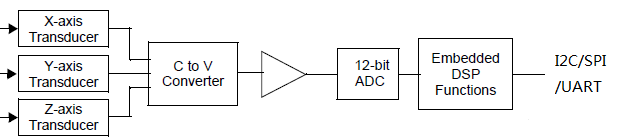【技术分享】九轴传感器之加速度计

“V^2 = Xout^2 + Yout^2 + Zout^2”（长方体：对角线^2 = 长^2 + 宽^2 + 高^2）

cos(Ax) = Xout / V    对应的     Ax = arcos(Xout / V)

cos(Ay) = Yout / V   对应的     Ay = arcos(Yout / V)

cos(Az) = Zout / V   对应的     Az = arcos(Zout / V)Xout = （(ADCx/4095) * Vref  – V0g) / Sens

Yout = （(ADCy/4095) * Vref  – V0g) / Sens

Zout = （(ADCz/4095) * Vref  – V0g) / Sens